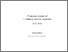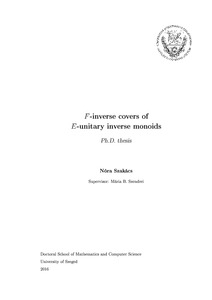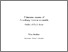# F-inverse covers of E-unitary inverse monoids

Szakács Nóra
F-inverse covers of E-unitary inverse monoids.
[Thesis] (Unpublished)Preview PDF (disszertáció) Download (907kB) | PreviewPreview PDF (tézis) Download (598kB) | PreviewPreview PDF (tézis) Download (604kB) | Preview

## Abstract in foreign language

The main motivation of the thesis is the so-called F-inverse cover problem. It asks whether every finite inverse monoid is a homomorphic image of a finite F-inverse monoid. The problem was originally formulated by Henckell and Rhodes, and a positive answer would have solved an important conjecture connected to the complexity theory of finite semigroups. The latter conjecture has been since proven , but the F-inverse cover problem has remained open. Significant progress has been made by Auinger and Szendrei in, where they have shown the F-inverse cover problem to be equivalent to a condition formulated by means of graphs and group varieties only. In the first part of the thesis, I investigate this condition, and establish that, if fixing the group variety, the graphs for which the condition is satisfied can be described using excluding minors. With this approach, as a main result, I give a full description of the graphs and group varieties satisfying it in the special case when the variety is Abelian. As far as the originally proposed problem goes, these results of do not say much. However, they motivated the following problem: describe inverse monoids S which have an F- inverse cover F such that F/\sigma is an extension of an Abelian group by S/\sigma - this we refer to as F being an F-inverse cover via the variety of Abelian groups. The results in my thesis and those of Auinger and Szendrei combined characterize such inverse monoids which are also Margolis-Meakin expansions. In Chapter 4 of the thesis, I generalize the framework introduced by Auinger and Szendrei so that it "works" for a much larger class of inverse monoids called finite-above E-unitary. In this framework, we obtain a sufficient condition for an E-unitary finite-above inverse monoid not to have an F-inverse cover via the variety of Abelian groups, formulated by means of the natural partial order and the least group congruence.

Item Type: Thesis (Doktori értekezés) Szakács Nóra E-unitér inverz monoidok F-inverz fedői Doctoral School of Mathematics Natural Sciences > Mathematics and Computer Sciences English 2016. May 11. 2890 3041230 https://doi.org/10.14232/phd.2890 2016. Apr. 11. 16:07 2020. Apr. 30. 08:15 B 6022 https://doktori.bibl.u-szeged.hu/id/eprint/2890 Defended.View Item